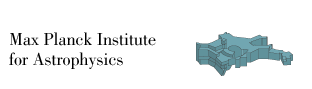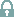# Identifying rotation with neutrinos in three dimensional core-collapse supernovae

Walk, L., Tamborra, I., Janka, H.-T., Summa, A. Phys.Rev. D98 (2018) no.12, 123001 [Paper 1], arXiv eprint 1807.02366.
Walk, L., Tamborra, I., Janka, H.-T., Summa, A. Phys.Rev. D100 (2019) no.6, 063018 [Paper 2], arXiv eprint 1901.06235.

## Movies

### Time evolution of the normalized electron neutrino lepton number

Time evolution of the electron neutrino lepton number (ELN) normalized to its average [(Nνe - Nν̅e)/<(Nνe - Nν̅e)>] on a Mollweide map of the progenitor's emission surface for the three 15 Msun supernova models with different rotational velocities. See Paper 2 for more details.

### Time evolution of the relative IceCube detection rate

Time evolution of the relative IceCube detection rate [(R-<R>)/<R>] on a sky-plot of observer directions for the three 15 Msun supernova models with different rotational velocities. The rate has been computed for the un-oscillated electron antineutrino flux (i.e., in the absence of flavor conversions). See Paper 1 for more details.

## Neutrino data

### Angle dependent neutrino emission properties

Luminosity (erg/s), mean energy (MeV), and mean squared energies (in MeV2) of νe, ν̅e, and νx for the three 15 Msun supernova models with different rotational velocities. The neutrino properties have been projected and recorded as seen by a distant observer, and mapped to a spherical grid consisting of angular zones in θ (inclination angle) and ϕ (azimuthal angle). The properties have been extracted from the simulation grid at a radius of 500 km. See Paper 2 for more details.

Each files contain post-bounce time (s), inclination angle (θ), azimuthal angle (ϕ), and the neutrino property at hand, recorded in four columns respectively. At each post-bounce time, the neutrino properties are recorded across all angular zones, ordered firstly by increasing θ values, and secondly across all ϕ coordinates.Download neutrino data for the non-rotating modelDownload neutrino data for the slowly rotating modelDownload neutrino data for the fast rotating model

The following (θ,ϕ) directions of the simulation grid correspond to the ones with strong, intermediate, and weak signal modulation (see Figs. 2-4 of Paper 2):

- non-rotating: strong: (3.0166, 0.19636), intermediate: (2.1242, 1.0889), weak: (1.6601, 1.6601)
- slowly rotating: strong: (0.58905, -2.374), intermediate: (0.66045, -0.33914), weak: (1.0174, 1.8743)
- fast rotating: strong: (3.088, -0.62474), intermediate: (1.5886, 0.94606), weak: (2.2312, 1.91)

### Spherically averaged neutrino emission properties

Spherically averaged luminosities (erg/s), mean energies (in MeV), and mean squared energies (in MeV2) [the energies are measured for the number flux] of the aforementioned models from Summa, A., et al., ApJ, 852, 28 (2018):Download neutrino data

For details about the supernova hydrodynamical simulations, see Summa, A., et al., ApJ, 852, 28 (2018).